• 交叉验证法

2020-12-28 15:16:30
交叉验证是用来观察模型的稳定性的一种方法，我们将数据划分为n份，依次使用其中一份作为测试集，其他n-1份作为训练集，多次计算模型的精确性来评估模型的平均准确程度。训练集和测试集的划分会干扰模型的结果，因此...

基本原理

交叉验证是用来观察模型的稳定性的一种方法，我们将数据划分为n份，依次使用其中一份作为测试集，其他n-1份作为训练集，多次计算模型的精确性来评估模型的平均准确程度。训练集和测试集的划分会干扰模型的结果，因此用交叉验证n次的结果求出的平均值，是对模型效果的一个更好的度量。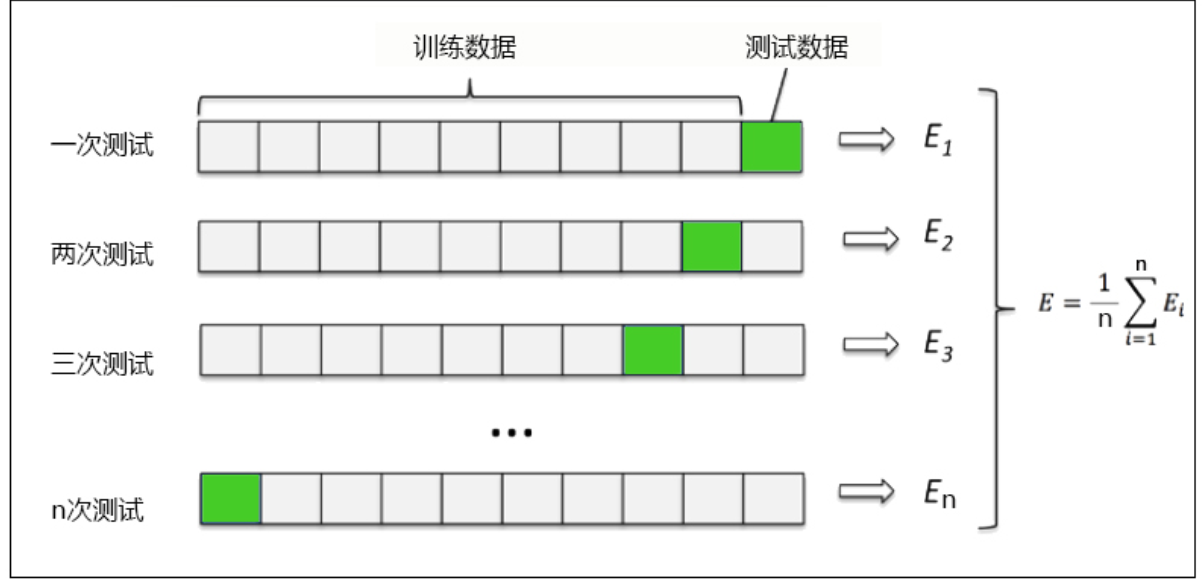实现代码及注释

from sklearn.model_selection import cross_val_score
from sklearn.tree import DecisionTreeRegressor

# 利用datasets中的波士顿房价数据集。
# 实例化一个树，与分类树类似。
regressor = DecisionTreeRegressor(random_state=0)
# 进行交叉验证。
# regressor模型评估器，可以是任何实例化过后的算法模型。
# boston.data数据集，不需要划分训练集与测试集，完整的数据集。
# boston_target 标签集
# cv = 10 交叉验证十次，通常为5 ，默认为5
# scoring返回衡量模型的结果，默认为R平方，neg_mean_squared_error为负均方误差

result = cross_val_score(regressor,
boston.data,
boston.target,
cv=10,
scoring = "neg_mean_squared_error")
print("交叉验证结果(负均方误差)：",result)

结果展示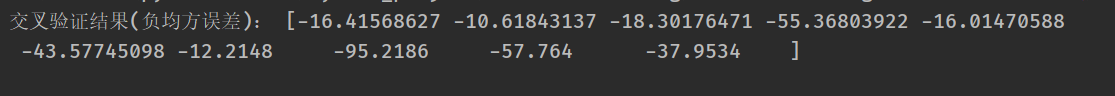虽然均方误差永远为正，但是sklearn当中使用均方误差作为评判标准时，却是计算”负均方误差“（neg_mean_squared_error）。这是因为sklearn在计算模型评估指标的时候，会考虑指标本身的性质，均方误差本身是一种误差，所以被sklearn划分为模型的一种损失(loss)，因此在sklearn当中，都以负数表示。真正的均方误差MSE的数值，其实就是neg_mean_squared_error去掉负号的数字。

展开全文机器学习 python 深度学习 人工智能 数据挖掘
• 上篇文章我们学习了模型评估的方法：留出法、自助法，接下来我们学习交叉验证法~ ****** 交叉验证 ****** 先将数据集D划分为k个大小相似的互斥子集，然后，每次用k-1个子集的并集作为训练集，剩下的一个子集作为测试...

上篇文章我们学习了模型评估的方法：留出法、自助法，接下来我们学习交叉验证法~

****** 交叉验证 ******

先将数据集D划分为k个大小相似的互斥子集，然后，每次用k-1个子集的并集作为训练集，剩下的一个子集作为测试集，这样就可以获得k组训练/测试集，从而可进行k次训练和测试，最终返回这k个测试结果的均值。k常用取值是10，即10折交叉验证，下面是10折示意图：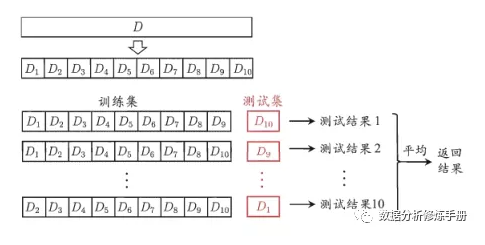注：

1. 分布一致性：每个子集都尽可能保持数据分布一致（分层采样）；
2. 多次随机、重复实验：由于数据集D划分为k个子集与留出法一样，同样存在多种划分方式，为了减少这种因样本划分不同而引入的误差，通常要随机划分p次数据集，最终结果是这p次k折交叉验证结果的均值；
3. 留一法：k=1，此时不受数据的随机划分的影响，但是当样本量较大时，计算成本会非常大。

****** 交叉验证分类 ******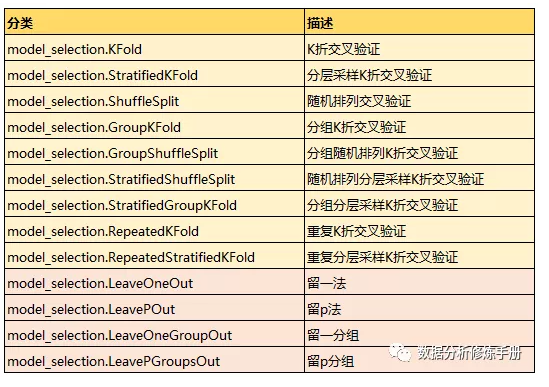****** 代码实现 ******

KFold（k折交叉验证）:

##提取数据
from pandas import DataFrame as df
########## KFold（K折交叉验证）
from sklearn.model_selection import KFold
kf = KFold(n_splits=6, shuffle=True)
'''
n_splits=6（默认5）：将数据集分成6个互斥子集，每次用5个子集数据作为训练集，1个子集为测试集，得到6个结果
shuffle=True（默认False）：每次划分前数据重新洗牌，每次的运行结果不同；shuffle=False：每次运行结果相同，相当于random_state=整数
可以单独设置shuffle，但是设置random_state必须设置shuffle=True
random_state=1（默认None）：随机数设置为1，使得每次运行的结果一致
'''
for train_index,test_index in kf.split(data_ori):
print(train_index,test_index)
x_train = data_ori.iloc[train_index,:]
x_test = data_ori.iloc[test_index,:]
y_train = data_ori['target'][train_index]
y_test = data_ori['target'][test_index]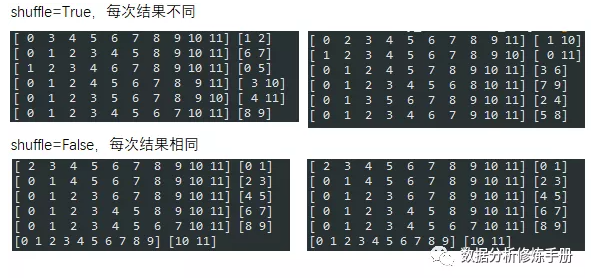StratifiedKFold（分层采样k折交叉验证）:

########## StratifiedKFold
from sklearn.model_selection import StratifiedKFold
strkf = StratifiedKFold(n_splits=6, shuffle=False)
'''
n_splits=6（默认5）：将数据集分成6个互斥子集，每次用5个子集数据作为训练集，1个子集为测试集，得到6个结果

shuffle=True（默认False）：每次划分前数据重新洗牌，每次的运行结果不同；shuffle=False：每次运行结果相同，相当于random_state=整数
random_state=1（默认None）：随机数设置为1，使得每次运行的结果一致
'''
for train_index,test_index in strkf.split(data_ori,data_ori['target']):
print(train_index,test_index)
x_train = data_ori.iloc[train_index,:]
x_test = data_ori.iloc[test_index,:]
y_train = data_ori['target'][train_index]
y_test = data_ori['target'][test_index]
## 数据集、训练集、测试集数据分布
data_pct = data_ori['target'].sum()/len(data_ori['target'])
y_train_pct = y_train.sum()/len(y_train)
y_test_pct = y_test.sum()/len(y_test)
print(data_pct, y_train_pct, y_test_pct)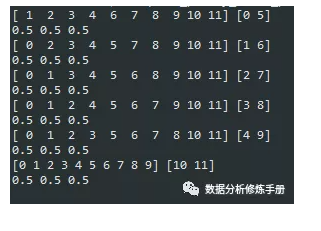ShuffleSplit（随机分组交叉验证）:

from sklearn.model_selection import ShuffleSplit
ShuffleS = ShuffleSplit(n_splits=6, test_size=0.4, random_state=1)
'''
将数据顺序打散之后再进行交叉验证
n_splits=6（默认10）：将数据集分成6个互斥子集，每次用5个子集数据作为训练集，1个子集为测试集，得到6个结果
test_size=0.4：测试集占比为40%
random_state=1（默认None）：随机数设置为1，使得每次运行的结果一致
'''
for train_index,test_index in ShuffleS.split(data_ori):
print(train_index,test_index)
x_train = data_ori.iloc[train_index,:]
x_test = data_ori.iloc[test_index,:]
y_train = data_ori['target'][train_index]
y_test = data_ori['target'][test_index]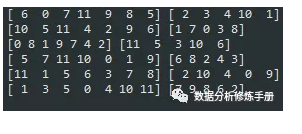GroupKFold（分组k折交叉验证）:

可以按照自己的需求来进行分组分组

########## GroupKFold（分组k折交叉验证）
from sklearn.model_selection import GroupKFold
groupkf = GroupKFold(n_splits=3)
groups = [1,1,1,1,1,1,2,2,2,2,3,3]
'''
* 同一组的样本不可能同时出现在同一折的测试集和训练集中。
* groups的分组数必须大于等于n_splits的值
'''
for train_index,test_index in groupkf.split(data_ori, groups=groups):
print(train_index,test_index)
x_train = data_ori.iloc[train_index,:]
x_test = data_ori.iloc[test_index,:]
y_train = data_ori['target'][train_index]
y_test = data_ori['target'][test_index]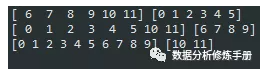RepeatedKFold（重复k折交叉验证）:

########## RepeatedKFold（重复n次k折交叉验证 ，每次重复有不同的随机性）
from sklearn.model_selection import RepeatedKFold
rptkf = RepeatedKFold(n_splits=6, n_repeats=2, random_state=1)
'''
n_splits=6（默认5）：将数据集分成6个互斥子集，每次用5个子集数据作为训练集，1个子集为测试集，得到6个结果n_repeats=2(默认10)：重复2次10折交叉验证
random_state=1（默认None）：随机数设置为1，使得每次运行的结果一致
'''
for train_index,test_index in rptkf.split(data_ori):
print(train_index,test_index)
x_train = data_ori.iloc[train_index,:]
x_test = data_ori.iloc[test_index,:]
y_train = data_ori['target'][train_index]
y_test = data_ori['target'][test_index]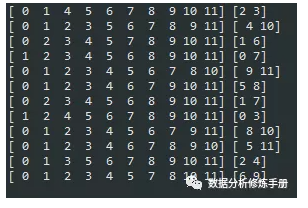RepeatedStratifiedKFold（重复分层采样k折交叉验证）:

########## RepeatedStratifiedKFold（重复n次分层采样的k折交叉验证 ，每次重复有不同的随机性）
from sklearn.model_selection import RepeatedStratifiedKFold
rptstrkf = RepeatedStratifiedKFold(n_splits=6, n_repeats=2, random_state=1)
'''
n_splits=6（默认5）：将数据集分成6个互斥子集，每次用5个子集数据作为训练集，1个子集为测试集，得到6个结果

n_repeats=2(默认10)：重复2次10折交叉验证
random_state=1（默认None）：随机数设置为1，使得每次运行的结果一致
'''
for train_index,test_index in rptstrkf.split(data_ori, data_ori['target']):
print(train_index,test_index)
x_train = data_ori.iloc[train_index,:]
x_test = data_ori.iloc[test_index,:]
y_train = data_ori['target'][train_index]
y_test = data_ori['target'][test_index]
## 数据集、训练集、测试集数据分布
data_pct = data_ori['target'].sum()/len(data_ori['target'])
y_train_pct = y_train.sum()/len(y_train)
y_test_pct = y_test.sum()/len(y_test)
print(data_pct, y_train_pct, y_test_pct)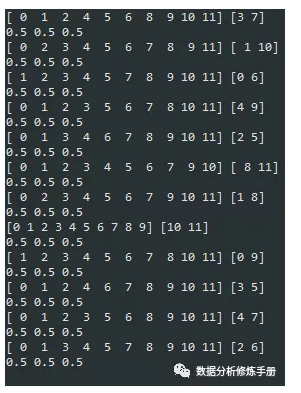留一法

########## 留一法
from sklearn.model_selection import LeaveOneOut
loo = LeaveOneOut()
for train_index,test_index in loo.split(data_ori):
print(train_index,test_index)
x_train = data_ori.iloc[train_index,:]
x_test = data_ori.iloc[test_index,:]
y_train = data_ori['target'][train_index]
y_test = data_ori['target'][test_index]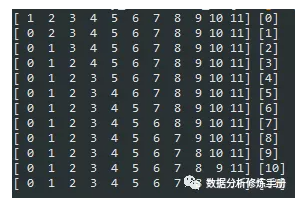留p法

########## 留P法
from sklearn.model_selection import LeavePOut
lpo = LeavePOut(p=1)
# p=1 :留1
for train_index,test_index in lpo.split(data_ori):
print(train_index,test_index)
x_train = data_ori.iloc[train_index,:]
x_test = data_ori.iloc[test_index,:]
y_train = data_ori['target'][train_index]
y_test = data_ori['target'][test_index]

GroupShuffleSplit、 StratifiedShuffleSplit、 StratifiedGroupKFold、 LeaveOneGroupOut、 LeavePGroupsOut 性质与上面类似，这里不再详细介绍啦~

========================================================================================

以上方法只是划分了数据集，而cross_val_score是根据模型计算交叉验证的结果，可以理解为cross_val_score中调用了KFold进行数据集划分

========================================================================================

cross_val_score

########## cross_val_score
## 提取数据
from pandas import DataFrame as df

## 建模
from sklearn.linear_model import LogisticRegression
lr = LogisticRegression(random_state=1)

##>> cv 为数值
from sklearn.model_selection import cross_val_score
scores = cross_val_score(estimator=lr, X=data_ori.iloc[:,:-1], y=data_ori['target'], cv=3, scoring='accuracy') #scoring（默认accuracy）：评价函数
print(scores, scores.mean()) # [0.93157895 0.96842105 0.92592593] 0.9419753086419753

##>> cv 为交叉验证方法
# KFold
from sklearn.model_selection import KFold
kf = KFold(n_splits=3, shuffle=True, random_state=1)
from sklearn.model_selection import cross_val_score
scores = cross_val_score(estimator=lr, X=data_ori.iloc[:,:-1], y=data_ori['target'], cv=kf, scoring='accuracy')
print(scores, scores.mean()) # [0.94210526 0.93157895 0.96825397] 0.9473127262600948

# ShuffleSplit
from sklearn.model_selection import ShuffleSplit
ShuffleS = ShuffleSplit(n_splits=3, random_state=1)
from sklearn.model_selection import cross_val_score
scores = cross_val_score(estimator=lr, X=data_ori.iloc[:,:-1], y=data_ori['target'], cv=ShuffleS, scoring='accuracy')
print(scores, scores.mean()) # [1.         0.96491228 0.96491228] 0.9766081871345028

cross_validate

########## cross_validate（多个评价指标）
from sklearn.model_selection import cross_validate
scoring = ['accuracy','f1']
scores = cross_validate(estimator=lr, X=data_ori.iloc[:,:-1], y=data_ori['target'], cv=3, scoring=scoring)
print(scores['test_accuracy'], scores['test_f1'])  # [0.93157895 0.96842105 0.92592593] [0.94693878 0.97520661 0.93965517]
print(scores['test_accuracy'].mean(), scores['test_f1'].mean()) # 0.9419753086419753 0.953933519831415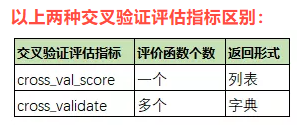GridSearchCV

########## GridSearchCV
##提取数据
import numpy as np
from pandas import DataFrame as df
from sklearn.model_selection import train_test_split
from sklearn.tree import DecisionTreeClassifier
from sklearn.model_selection import GridSearchCV

##提取数据
x_train, x_test, y_train, y_test = train_test_split(data_ori.iloc[:,:-1], data_ori['target'], test_size=0.2, random_state=1)

## GridSearchCV
DT = DecisionTreeClassifier()
param = {'max_depth':[3,4,5], 'min_samples_leaf':[2,3,4], 'min_impurity_decrease':[0.1,0.2]}
DTGrid = GridSearchCV(estimator=DT, param_grid=param, cv=3, scoring='accuracy')
DTGrid.fit(x_train,y_train)

## 交叉结果表
means = DTGrid.cv_results_['mean_test_score']
stds = DTGrid.cv_results_['std_test_score']
params = DTGrid.cv_results_['params']
for i, j,k in zip(means,stds,params):
print('mean:{:.6f}'.format(i), 'std:{:.6f}'.format(j), 'param:',k)

## 最优参数及分数
print(DTGrid.best_params_) # 最优参数{'max_depth': 3, 'min_impurity_decrease': 0.1, 'min_samples_leaf': 2}
print(DTGrid.best_score_) # 最优参数对应的分数（默认使用的accuracy）0.9033490182409666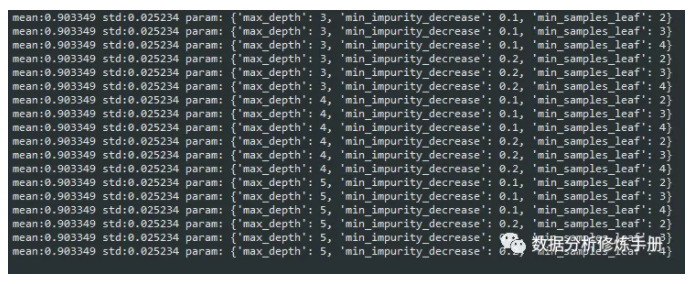详细内容请看我公众号~
【模型评估_方法_交叉验证法】

展开全文python 机器学习 人工智能
• 本帖最后由 小新1234 于 2019-12-28 10:45 编辑[有偿]网络上下载了一个代码，用K折交叉验证法验证贝叶斯网络的准确性，代码不会调整，有没有大神帮忙看看哪里有问题clear;clc;ex=importdata('test.txt');%读入文件X=...

本帖最后由 小新1234 于 2019-12-28 10:45 编辑

[有偿]网络上下载了一个代码，用K折交叉验证法验证贝叶斯网络的准确性，代码不会调整，有没有大神帮忙看看哪里有问题

clear;

clc;

ex=importdata('test.txt');  %读入文件

X=ex.data;

[m,n]=size(ex.textdata);  %数据大小

Y=zeros(m);

for i=1:m

if strcmp(ex.textdata(i),'A')==1

Y(i)=1;

elseif strcmp(ex.textdata(i),'B')==1

Y(i)=2;

elseif strcmp(ex.textdata(i),'C')==1

Y(i)=3;

elseif strcmp(ex.textdata(i),'D')==1

Y(i)=4;

else Y(i)=5;

end

end

%朴素贝叶斯算法实现分类问题(五类y=1,y=2,y=3，y=4,y=5)

%我们把所有数字序号末尾为1的留作测试集，其他未训练集

% m=4113;   %样本总数

% m1=562;  %训练集样本数量

% m2=63;  %测试集样本数量

%5折5次交叉验证

indices = crossvalind('Kfold',m,5); %产生5个fold，即indices里有等比例的1-5

accuracy = 0;

for i=1:5

test=(indices==i); %逻辑判断，每次循环选取一个fold作为测试集

train=~test; %取test的补集作为训练集，即剩下的4个fold

data_train=X(train,; %以上得到的数都为逻辑值，用与样本集的选取

label_train=Y(train,; %label为样本类别标签，同样选取相应的训练集

data_test=X(test,; %同理选取测试集的样本和标签

label_test=Y(test,;

[l1,l3] = size(label_train);

[l2,l4] = size(label_test);

%5类样本数量分别记为count1,count2,count3,4,5

count1=0;

count2=0;

count3=0;

count4=0;

count5=0;

%count_1(i,j)表示在第一类(y=1)的情况下，第i个属性是j的样本个数

count_1=zeros(4,5);

%count_2(i,j)表示在第二类(y=2)的情况下，第i个属性是j的样本个数

count_2=zeros(4,5);

%count_3(i,j)表示在第三类(y=3)的情况下，第i个属性是j的样本个数

count_3=zeros(4,5);

count_4=zeros(4,5);

count_5=zeros(4,5);

ii=1;%用来标识测试集的序号

for i=1:l1

x=data_train(i,;

if label_train(i)==1

count1=count1+1;

for j=1:4    %指示第j个属性

for k=1:5    %第j个属性为哪个值

if x(j)==k

count_1(j,k)=count_1(j,k)+1;

break;

end

end

end

elseif label_train(i)==2

count2=count2+1;

for j=1:4   %指示第j个属性

for k=1:5    %第j个属性为哪个值

if x(j)==k

count_2(j,k)=count_2(j,k)+1;

break;

end

end

end

elseif label_train(i)==3

count3=count3+1;

for j=1:4    %指示第j个属性

for k=1:5    %第j个属性为哪个值

if x(j)==k

count_3(j,k)=count_3(j,k)+1;

break;

end

end

end

elseif label_train(i)==4

count4=count4+1;

for j=1:4    %指示第j个属性

for k=1:5    %第j个属性为哪个值

if x(j)==k

count_4(j,k)=count_4(j,k)+1;

break;

end

end

end

elseif label_train(i)==5

count5=count5+1;

for j=1:4    %指示第j个属性

for k=1:5    %第j个属性为哪个值

if x(j)==k

count_5(j,k)=count_5(j,k)+1;

break;

end

end

end

end

%分别计算三类概率y1=p(y=1)、y2=p(y=2)、y3=p(y=3)的估计值

y1=count1/l1;

y2=count2/l1;

y3=count3/l1;

y4=count4/l1;

y5=count5/l1;

%y_1(i,j)表示在第一类(y=1)的情况下，第i个属性取值为j的概率估计值

%y_2(i,j)表示在第二类(y=2)的情况下，第i个属性取值为j的概率估计值

%y_3(i,j)表示在第三类(y=3)的情况下，第i个属性取值为j的概率估计值

for i=1:4

for j=1:5

y_1(i,j)=count_1(i,j)/count1;

y_2(i,j)=count_2(i,j)/count2;

y_3(i,j)=count_3(i,j)/count3;

y_4(i,j)=count_4(i,j)/count4;

y_5(i,j)=count_5(i,j)/count5;

end

end

end

%做预测,p1,p2,p3分别表示输入值xx为第1，2，3类的概率

cc=0;   %用cc表示正确分类的样本

for i=1:l2

xx=data_test(i,;

yy=label_test(i);

p1=y1*y_1(1,xx(1))*y_1(2,xx(2))*y_1(3,xx(3))*y_1(4,xx(4));——(这一步就运行不下去了)

p2=y2*y_2(1,xx(1))*y_2(2,xx(2))*y_2(3,xx(3))*y_2(4,xx(4));

p3=y3*y_3(1,xx(1))*y_3(2,xx(2))*y_3(3,xx(3))*y_3(4,xx(4));

p4=y4*y_4(1,xx(1))*y_4(2,xx(2))*y_4(3,xx(3))*y_4(4,xx(4));

p5=y5*y_5(1,xx(1))*y_5(2,xx(2))*y_5(3,xx(3))*y_5(4,xx(4));

%下面分别输出三类的概率

%ans1=p1/(p1+p2+p3+p4+p5)

%ans2=p2/(p1+p2+p3+p4+p5)

%ans3=p3/(p1+p2+p3+p4+p5)

%ans4=p4/(p1+p2+p3+p4+p5)

%ans4=p5/(p1+p2+p3+p4+p5)

if p1>p2&&p1>p3 ——(这一步没有改，我的是五类)

if yy==1

cc=cc+1;

end

end

if p2>p1&&p2>p3

if yy==2

cc=cc+1;

end

end

if p3>p1&&p3>p2

if yy==3

cc=cc+1;

end

end

end

%拿训练集做测试集，得到的正确率

accur_per = cc/l2

accuracy = accuracy + accur_per;

end

accur_ave = accuracy/102019-12-27 22:00 上传

点击文件名下载附件

293.21 KB, 下载次数: 6

展开全文• 其实在机器学习中还有其他划分数据集的方法，可以在本身数据总量就比较小时使模型达到很好的效果，我们今天介绍的交叉验证法就是比较常用的方法，它在我们将要介绍的集成学习的Stacking算法中经常使用到。...

本文作者：王 歌

文字编辑：戴 雯

技术总编：张 邯Python云端培训课程火热招生中~重大通知！！！爬虫俱乐部于2020年7月11日至14日在线上举行为期四天的Stata编程技术定制培训，招生工作已经圆满结束啦！！！

另外，应广大学员需求，爬虫俱乐部将于2020年7月25日至28日在线上举行Python编程技术训练营，本次培训采用理论与案例相结合的方式，帮助大家在掌握Python基本思想的基础上，学习科学计算技术与网络数据抓取技术，详情可点击《Python云端培训课程开始报名~》，或点击文末阅读原文直接提交报名信息呦~导读前面我们在举例时，通常是将所使用的数据集按照75%和25%的比例划分为训练集和测试集，这主要是为了我们举例方便，同时划分后的数据量也依然符合大样本的要求。其实在机器学习中还有其他划分数据集的方法，可以在本身数据总量就比较小时使模型达到很好的效果，我们今天介绍的交叉验证法就是比较常用的方法，它在我们将要介绍的集成学习的Stacking算法中经常使用到。

1方法介绍我们往往会得到多个模型，而最终选择的模型必定是泛化能力强，也就是在未知的新数据上效果最好的模型，因此我们在训练模型前就要将我们所掌握的数据进行划分，严格来说一般会划分为训练集、验证集和测试集，在训练集上进行训练模型，在验证集上试验效果、调整参数设置，在测试集上进行最终的测试。为了保证最终的效果，这三个集合不能有交集，常见的比例是8:1:1。当然，通常我们只有训练集和测试集也是可以的，前面我们使用的样例数据集只有几百个，因此也没有划分验证集。我们所使用的train_test_split属于留出法，也就是随机将一部分数据作为训练集，剩下的作为测试集。但对样本信息的利用往往不充分，并且需要的样本量较大。如果我们本身样本量有限，并且想充分利用数据集中的信息，我们可以采用交叉验证法。交叉验证法是将数据集划分为k个大小相似的互斥子集，并在划分时保持数据分布的一致性，每次用k-1个子集的并集作为训练集，剩余的做测试集，进行k次训练，最后取k次结果的均值。该方法依赖于k值的选取，通常取10，因此也称为k折交叉验证(k-fold-cross-validation)，当k=1时称为留一法(Leave-One-Out)。由于留一法在样本量大时计算量较大，所以主要用于样本量比较少的情况。在实际应用中，我们只进行一次交叉验证可能是不够的，而要进行多次，称为p次k折交叉验证，一般取p=k=10。以上方法在sklearn中都有相应的类来实现，我们下面来看一下。

2程序实现

我们这里依然使用的是鸢尾花的数据，同时使用Logistic回归训练模型。在sklearn中，通常使用 cross_val_predict实现k折交叉验证，它返回的是一个使用交叉验证以后的输出值，若要返回准确度评分，可以使用 cross_val_score。两者参数相同，第一个参数为所使用的分类器，第二个和第三个参数分别是属性值和标签值，最后一个参数 cv确定折数。我们这里进行5折的交叉验证，程序如下：from sklearn.datasets import load_irisfrom sklearn.linear_model import LogisticRegressionfrom sklearn.model_selection import cross_val_score, cross_val_predictiris_sample = load_iris()x = iris_sample.datay = iris_sample.targetlrclf = LogisticRegression()predicted = cross_val_predict(lrclf, x, y, cv=5) #计算预测值print('5折交叉验证预测值：', predicted)scores = cross_val_score(lrclf, x, y, cv=5) #计算模型的评分情况print('评分：', scores)print('准确度：', metrics.accuracy_score(predicted, y)) #计算评分的均值结果如下：若使用留一法，则要使用 LeaveOneOut类，没有参数需要设置。具体程序如下：from sklearn.model_selection import LeaveOneOutloo = LeaveOneOut()scores = cross_val_score(lrclf, x, y, cv=loo)predicted = cross_val_predict(lrclf, x, y, cv=loo)print('留一法预测值：', predicted)scores = cross_val_score(lrclf, x, y, cv=loo)print('评分：', scores)print('准确度：', metrics.accuracy_score(predicted, y)结果如下：假设进行5次5折交叉验证，我们使用 RepeatedKFold类，有三个参数：

(1) n_split表示要划分的折数；

(2) n_repeats表示重复几次；

(3) random_state设置随机种子。程序如下：from sklearn.model_selection import RepeatedKFoldkf = RepeatedKFold(n_splits=5, n_repeats=5, random_state=0) #种子设为0predicted = cross_val_predict(lrclf, x, y, cv=5)print('5次5折交叉验证预测值：', predicted)scores = cross_val_score(lrclf, x, y, cv=kf)print('评分：', scores)print('准确度：', metrics.accuracy_score(predicted, y))结果如下：在sklearn中还提供了许多其它交叉验证的类，比如使用 ShuffleSplit类可以随机的把数据打乱，然后分为训练集和测试集；对于时间序列的数据，可以使用 TimeSeriesSplit；若要实现分层抽样式的交叉验证，可以使用 StratifiedKFold；分层随机划分可以使用 StratifiedShuffleSplit，等等，大家可以根据自己的需要来选择合适的交叉验证方式。以上就是本期推文的全部内容啦，感兴趣的小伙伴快动手试试吧~对我们的推文累计打赏超过1000元，我们即可给您开具发票，发票类别为“咨询费”。用心做事，不负您的支持！往期推文推荐关于我们微信公众号“Stata and Python数据分析”分享实用的stata、python等软件的数据处理知识，欢迎转载、打赏。我们是由李春涛教授领导下的研究生及本科生组成的大数据处理和分析团队。此外，欢迎大家踊跃投稿，介绍一些关于stata和python的数据处理和分析技巧。投稿邮箱：statatraining@163.com投稿要求：1)必须原创，禁止抄袭；2)必须准确，详细，有例子，有截图；注意事项：1)所有投稿都会经过本公众号运营团队成员的审核，审核通过才可录用，一经录用，会在该推文里为作者署名，并有赏金分成。2)邮件请注明投稿，邮件名称为“投稿+推文名称”。3)应广大读者要求，现开通有偿问答服务，如果大家遇到有关数据处理、分析等问题，可以在公众号中提出，只需支付少量赏金，我们会在后期的推文里给予解答。展开全文• 在训练数据的过程或者参加数据比赛的时候，常常会遇到数据量不够大的情况，在一次比赛过程我学到一个小技巧—K折交叉验证法(k-fold CrossValidation)，下面和大家分享一下。1 变形前的K折在遇到K折交叉验证之前，...
• 设置验证集的作用： 首先用训练集合多个正则化超参数训练多个模型，选择在验证集上有最佳性能的模型和超参数。 最后用测试集得到结果。 如果没有验证集，直接在测试集上调至...下面交叉验证的讲法更容易理解： ...
• 数据集划分 训练集 验证集 测试集 ...交叉验证 1.留一验证法 把一个大的数据集分为k个小数据集，k等于数据集中数据的个数，每次只使用一个作为测试集，剩下的全部作为训练集，这种方法得出的结果与训练整个测试集的期深度学习 python
• # 留出 from sklearn.model_selection import train_test_split import pandas as pd df = pd.read_csv('iris.csv') X = df.iloc[:,1:5] Y = df.iloc[:,-1] train_X, test_X, train_Y, test_Y = train_test_...python 机器学习
• 显然，交叉验证法评估结果的稳定性和保真性在很大程度上取决于k的取值，为强调这一点，通常把交叉验证法称为“k折交叉验证”。 9、分析偏差和方差的含义。 偏差度量了学习算法的期望预测与真实结果的偏离程度，即刻...机器学习 人工智能 sklearn
• """ @author: JacksonKim @filename: k_fold_cross_validation.py @start: 2021/1/29 @end: 2021/1/29 """ import numpy as np ...3. 交叉验证法评估结果的稳定性和保真性很大程度上取决于k的取值机器学习 python 决策树 深度学习
• fold Cross-Validation用于模型评估) 交叉验证法(cross validation)/k折交叉验证(k-fold cross validation)：将数据集D划分成k个大小相似的互斥子集，每个子集Di都尽量保持数据分布的一致性，即从D中通过分层采样...K折交叉验证 过拟合 机器学习
• 交叉验证(Cross-validation)主要用于建模应用中，例如PCR （主成分回归）、PLS （偏最小二乘）回归建模中。在给定的建模样本中，拿出大部分样本进行建模型，留小部分样本用刚建立的模型进行预报，并求这小部分样本的...python 机器学习
• 模型评估(Model Evaluation) 1.测试集(testing set) 测试集(testing set)： 通常，我们可通过实验测验来对学习器的泛化误差进行评估并进而做出选择，为此，需要一个“测试集”来测试学习器对新样本的判别能力。...
• 留出法和交叉验证

千次阅读 2021-04-26 15:15:37
评估方法 主要分三种：留出法(分一次 互斥集)交叉验证法(分多次 对k折形成多次互斥集)自助法(有放回抽样)留出法代码如下：function [X_train, y_train, X_test, y_test] = split_train_test(X, y, k, ratio)%SPLIT_...
• 2.把整个数据集划分成为训练集、验证集和测试集(一般的比例是6:2:2，参考NG《ML》)。其中训练集用于训练出模型的参数(除超参数外的惨呼)，验证集用于模型优化，即寻找最优的超参数，测试集用于评估最终模型的泛化...java 交叉验证
• 交叉验证(Cross Validation,CV)是用来验证分类器的性能一种统计分析方法，基本思想是把在某种意义下将原始数据(dataset)进行分组,一部分做为训练集(train set),另一部分做为验证集(validation set)。首先用训练集对...
• 关于逻辑回归模型的验证，解释了使用留出划分数据的不稳定性，以及如何使用n折交叉验证得到AUC值的均值，内含有循环绘制标准ROC曲线的方法。一个模型很重要的是其在新样本的预测能力，因此只在原数据集上回测检验...python 机器学习 逻辑回归
• 为什么要用交叉验证

千次阅读 2020-12-24 21:36:12
本文结构：什么是交叉验证法？为什么用交叉验证法？主要有哪些方法？优缺点？各方法应用举例？什么是交叉验证法？它的基本思想就是将原始数据(dataset)进行分组，一部分做为训练集来训练模型，另一部分做为测试集来...
• 十折交叉验证(1)英文名叫做10-fold cross-validation，用来测试算法准确性，是常用的测试方法。(2)将数据集分成十份，轮流将其中9份作为训练数据，1份作为测试数据，进行试验。每次试验都会得出相应的正确率(或差错...
• 从csv文件中读取数据，由于样本量只有30，因此选择采用留一交叉验证方法，实验了一圈，发现一些问题在网上没有找到很好地解决办法，特记录如下，方便其他人使用。 主要代码如下：使用LeaveOneOut()将数据的索引分成...
• 机器学习中这三种数据集合非常容易弄混，特别是验证集和测试集，这篇笔记写下我对它们三个的理解以及在实践中是如何进行划分的。 训练集 这个是最好理解的，用来训练模型内参数的数据集，Classfier直接根据训练集...
• 3.3 选择两个UCI数据集，比较10折交叉验证法和留一法所估计出的对率回归的错误率 数据集：鸢尾花数据集 数据集属性信息： 1.萼片长度（以厘米计） 2.萼片宽度（以厘米计） 3.花瓣长度（以厘米计） 4.花瓣宽度（以...机器学习
• 五折交叉验证： 把数据平均分成5等份，每次实验拿一份做测试，其余用做训练。实验5次求平均值。如上图，第一次实验拿第一份做测试集，其余作为训练集。第二次实验拿第二份做测试集，其余做训练集。依此类推~ 但是，...
• python中sklearnk折交叉验证发布时间：2018-06-10 11:09,浏览次数：492, 标签：pythonsklearnk1.模型验证回顾进行模型验证的一个重要目的是要选出一个最合适的模型，对于监督学习而言，我们希望模型对于未知数据的...
• function [cvmse,gam_best,sig2_best] = crossvalidate(Xtrain,Ytrain,gam_min,gam_max,sig2_min,sig2_max,k)%%%%%%%%%%%%%%%%子程序_交叉验证程序%%%函数输入：Xtrain-训练集的输入，Ytrain-训练集的输出，gam_min-...
• 如果您使用kNN和交叉验证来查找最佳k，则应在训练/测试中拆分数据集，然后在训练/验证集中拆分训练集 . 然后使用简化训练集训练并使用验证集来确定哪个k是最佳的 . 在二元分类的情况下，例如面对非面，错误率通常...
• Python中sklearn实现交叉验证一、概述1.1 交叉验证的含义与作用1.2 交叉验证的分类二、交叉验证实例分析2.1 留一实例2.2 留p实例2.3 k折交叉验证（Standard Cross Validation）实例2.4 随机分配交叉验证...python 机器学习 数据分析
• k折交叉验证(R语言)

千次阅读 2020-12-24 21:35:27
”k折交叉验证K折交叉验证(k-foldcross-validation)首先将所有数据分割成K个子样本，不重复的选取其中一个子样本作为测试集，其他K-1个样本用来训练。共重复K次，平均K次的结果或者使用其它指标，最终得到一个单一...
• 笔记下面是代码，注释一般都能看懂的。%datas为读入的数据集 labels为读入的标签%规范化数据[datas_normal] = premnmx(datas) ;...%交叉验证,使用十折交叉验证 Kfold%indices为 m 行一列数据，表示每个训练样本属于...
• 我知道这个问题已经过时了，但如果有人想做类似的事情，请扩展一下ahmedhosny's答案：新的tensorflow数据集API能够使用python生成器创建数据集对象，因此除了scikit learn的KFold选项外，还可以使用KFold.split()......